Maths
Study Material

# Integration Formula

Integration formulas can be applied for the integration of various different functions such as algebraic expressions, trigonometric functions, inverse trigonometric functions, logarithmic and exponential functions.

3 minutes longHesap Oluştur

Got stuck on homework? Get your step-by-step solutions from real tutors in minutes! 24/7. Unlimited.

Integration is a fundamental operation of calculus. It has numerous uses in mathematics from calculation of volume of solid, area of arbitrary shape and length of a curve etc. Integration can be considered the reverse process of differentiation and can be called Inverse Differentiation. Here, we are going to learn about all integration formula.

## Integration Formula

Integration formulas can be applied for the integration of various different functions such as algebraic expressions, trigonometric functions, inverse trigonometric functions, logarithmic and exponential functions.

## Basic Integration Formulas

Here, we will learn about the most basic integration formula in indefinite integration.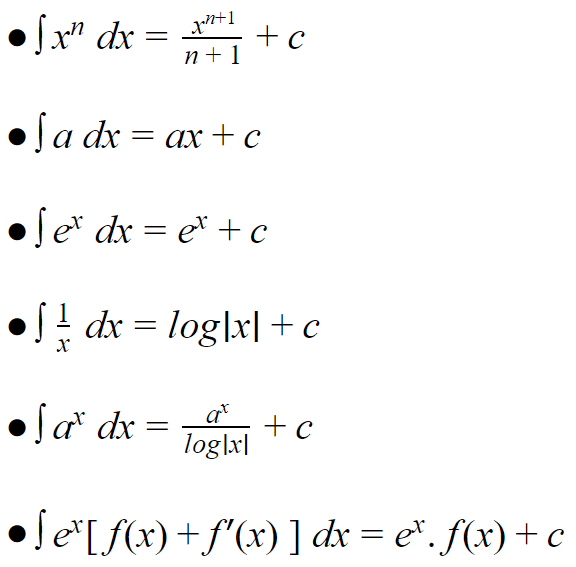These are the basic integration formulas used in indefinite integration to solve numericals.

## Trigonometric Functions – Integration Formula List

Trigonometric functions can be defined as the functions of an angle of a triangle. The basic trigonometric functions are sine, cosine, tangent, cotangent, secant and cosecant. To learn more about Trigonometry and its formulas check out Trigonometry Formula(s).

Here, we will learn about the formula of integration of these functions.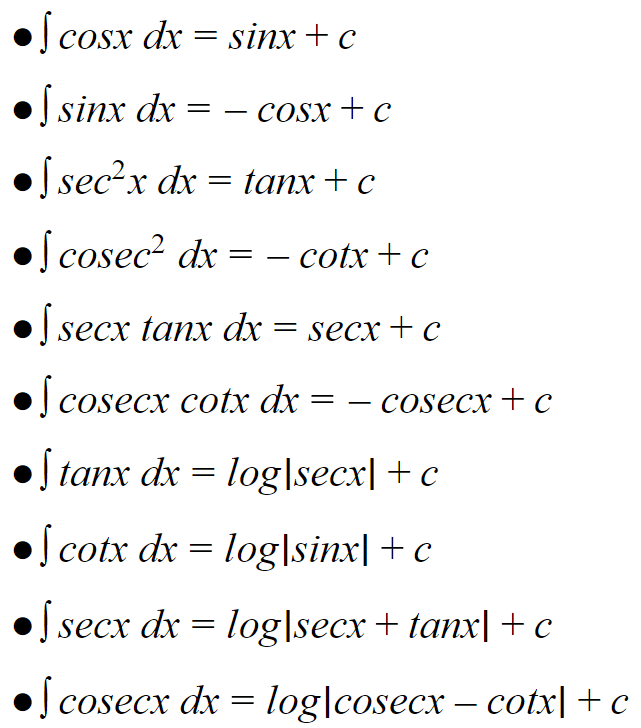## Inverse Trigonometric Functions – Integration Formulas List

Inverse trigonometric functions are basically the inverse of the basic trigonometric functions i.e. inverse of sine, cosine, tangent, cotangent, secant, and cosecant functions.

Integration of these inverse trigonometric functions are given below: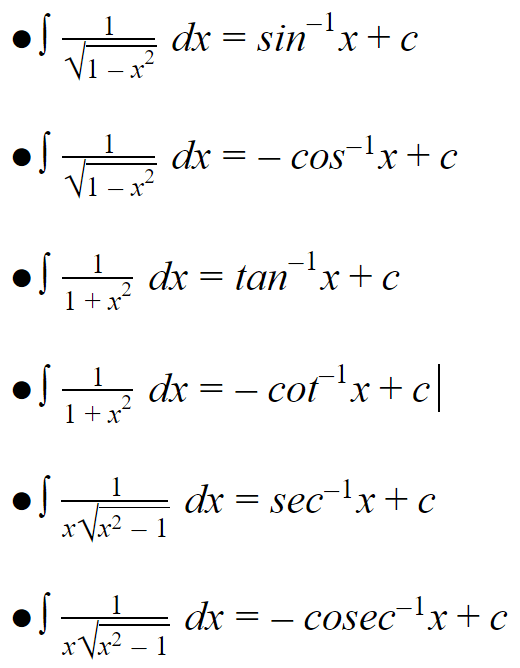## Special Integration Formula

These are some of the special integration formulas which are very important in higher level exams.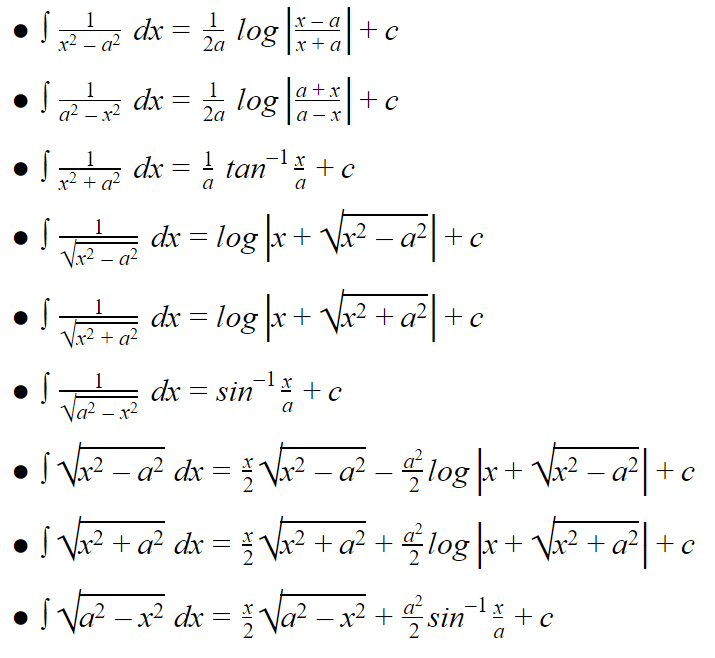All these are important integration formulas for class 12.

## Integration by Parts

The integration by parts formula is calculated as :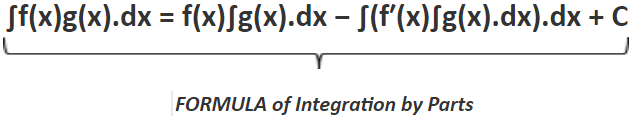Thus, it can be seen that the expression of the product rule of integration is divided in two parts; the first term consists of the function f(x) and the integral of the function g(x) and the second term constitutes of the whole integration of differentiation of the function f(x) and the integral of the function g(x). Along with which, there exists an integration constant also.

To learn about integration by parts and it’s rule check out Integration by Parts.

## Picked For You

Furthermore, these are some topics which might interest you: